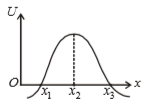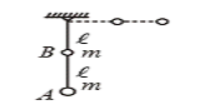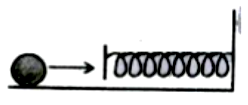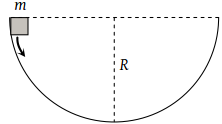A steel wire can withstand a load up to 2940 N. A load of 150 kg is suspended from a rigid support. The maximum angle through which the wire can be displaced from the mean position, so that the wire does not break when the load passes through the position of equilibrium, is (2008 E)

(1) 30$°$

(2) 60$°$

(3) 80$°$

(4) 85$°$

Concept Questions :-

Work-Energy theorem
High Yielding Test Series + Question Bank - NEET 2020

Difficulty Level:

A body is thrown vertically up with certain initial velocity, the potential and kinetic energies of the body are equal at a point P in its path. If the same body is thrown with double the velocity upwards, the ratio of potential and kinetic energies of the body when it crosses the same point, is

1. 1:1

2. 1:4

3. 1:7

4. 1:8

Concept Questions :-

Gravitational Potential energy
High Yielding Test Series + Question Bank - NEET 2020

Difficulty Level:

A body is displaced from (0,0) to (1m,1m) along the path x=y by a force $F=\left({x}^{2}\stackrel{^}{j}+y\stackrel{^}{i}\right)N$. The work done by this force will be :

1. $\frac{4}{3}J$

2. $\frac{5}{6}J$

3. $\frac{3}{2}J$

4. $\frac{7}{5}J$

Concept Questions :-

Work done by variable force
High Yielding Test Series + Question Bank - NEET 2020

Difficulty Level:

In the figure shown, the potential energy U of a particle is plotted against its position 'x' from the origin. Then which of the following statement is correct?1. ${\mathrm{x}}_{1}$ is in stable equilibrium

2. ${\mathrm{x}}_{2}$ is in stable equilibrium

3. ${\mathrm{x}}_{3}$ is in stable equilibrium

4. none of these

Concept Questions :-

Potential energy: Relation with force
High Yielding Test Series + Question Bank - NEET 2020

Difficulty Level:

A weightless rod of length 2l carries two equal mass 'm', one tied at lower end A and the other at the middle of the rod at B. The rod can rotate in a vertical plane about a fixed horizontal axis passing through C. The rod is released from rest in the horizontal position. The speed of the mass B at the instant rod, become vertical is:(1) $\sqrt{\frac{3gl}{5}}$

(2) $\sqrt{\frac{4gl}{5}}$

(3) $\sqrt{\frac{6gl}{5}}$

(4) $\sqrt{\frac{7gl}{5}}$

Concept Questions :-

Work-Energy theorem
High Yielding Test Series + Question Bank - NEET 2020

Difficulty Level:

A force F is applied on a body which moves with a velocity v in the direction of the force, then the power will be

1.  ${\mathrm{Fv}}^{2}$

2.  Fv

3.  $\mathrm{F}/{\mathrm{v}}^{2}$

4.  F/v

High Yielding Test Series + Question Bank - NEET 2020

Difficulty Level:

Potential energy (U) related to coordinates is given by U = 3(x + y). Work was done when the particle is going from (0, 0), (2, 3) is:

1.  15 J

2.  -15 J

3.  12 J

4.  10 J

High Yielding Test Series + Question Bank - NEET 2020

Difficulty Level:

A mass of 0.5kg moving with a speed of 1.5 m/s on a horizontal smooth surface collides with a nearly weightless spring of force constant k = 50 N/m. The maximum compression of the spring would be1.  0.5 m

2.  0.15 m

3.  0.12 m

4.  1.5 m

High Yielding Test Series + Question Bank - NEET 2020

Difficulty Level:

A mass m slips along the wall of a semispherical surface of radius R. The velocity at the bottom of the surface is [ MP PMT 1993](1) $\sqrt{Rg}$

(2) $\sqrt{2Rg}$

(3) $2\sqrt{\pi Rg}$

(4) $\sqrt{\pi Rg}$

Concept Questions :-

Gravitational Potential energy
High Yielding Test Series + Question Bank - NEET 2020

Difficulty Level:

Three different objects of masses and m3 are allowed to fall from rest and from the same point ‘O’ along three different frictionless paths. The speeds of the three objects, on reaching the ground, will be in the ratio of

(1) ${m}_{1}:{m}_{2}:{m}_{3}$

(2) ${m}_{1}:2{m}_{2}:3{m}_{3}$

(3) 1 : 1 : 1

(4) $\frac{1}{{m}_{1}}:\frac{1}{{m}_{2}}:\frac{1}{{m}_{3}}$

Concept Questions :-

Gravitational Potential energy